Maths
Study Material

# Mean Value Theorem

Mean Value Theorem, a crucial concept and an important theorem, is one of the most useful and efficient tools in the calculus (in differential as well as integral calculus).

8 minutes long
Posted by Kunduz Tutor, 1/11/2021Hesap Oluştur

Got stuck on homework? Get your step-by-step solutions from real tutors in minutes! 24/7. Unlimited.

Mean Value Theorem, a crucial concept and an important theorem, is one of the most useful and efficient tools in the calculus (in differential as well as integral calculus). It plays a significant role in the analysis of the behaviour of the functions.

We will be discussing about the mean value theorem formula, the proof of MVT along with some of the mean value theorem examples. Moreover, we shall look about the MVT(s) and acquire more knowledge regarding those concepts.

Author – Ojasvi Chaplot

## Introduction

All the mean value theorem(s) are defined on the smooth curves (which are continuous and differentiable). According to the mean value theorems, it is stated that the average rate of change is equal to the instantaneous rate of change at some given point of time.

The Mean Value Theorem can also be abbreviated as MVT.

## Mean Value Theorem Formula

The statement of MVT states that for a curve F(x) passing through two given points (a, F(a)) and (b, F(b)), there exists at least one point (c, F(c)) on the curve where the tangent is parallel to the secant passing through these two given points.

The mean value theorem is defined as, for a function F(x): [a, b] → R, such that:

• The function F(x) is continuous across the closed interval [a, b].
• The function F(x) is differentiable across the open interval (a, b).

Then, there exists a real value c in (a, b)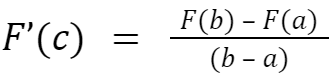where, c ∈ (a, b); implies that a < c < b. And, points a and b are the boundary values.

Here, with the help of MVT, we have proved that the tangent at the point c is parallel to the secant (or chord) passing through the points (a, F(a)) and (b, F(b)). Hence, this MVT is used to prove a statement across a closed interval.

Further, Rolle’s Mean Value Theorem is derived from this MVT and Lagrange’s Mean Value Theorem is an extended mean value theorem.

## Proof of Mean Value Theorem

Statement: The mean value theorem states that if a function F is continuous over the closed interval [a, b], and is differentiable over the open interval (a, b), then there exists at least one point c in the interval (a, b) such that F(c) is the average rate of change of the function over [a, b] and it is parallel to the secant line over [a, b].

Proof: Let G(x) be the secant line to F(x) passing through (a, F(a)) and (b, F(b)). As we know that the equation of the line can be written as (y – y1) = m (x – x1). So, the equation of the secant (or chord) is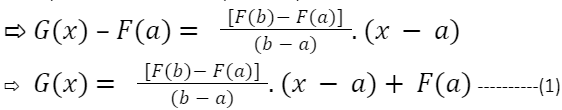Let H(x) = F(x) – G(x)

From equation (1), on substituting the value of G(x), we get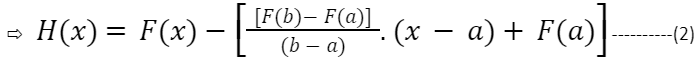Since, H(a) = 0 and H(b) = 0

? H(a) = H(b) = 0

And, H(x) is continuous on [a, b] and differentiable on (a, b).

Therefore, on applying the Rolle’s MVT

So, there exists some value x = c in (a, b) such that H'(c) = 0.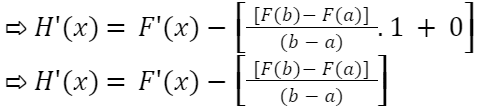At x = c, H'(x) = 0.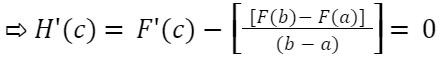Thus,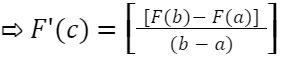Hence, the MVT is proved.

Also note that the result of the MVT may not hold if the function is not continuous or differentiable, even at a single point.

## Cauchy Mean Value Theorem

Let F and G be two real-valued functions defined on [a, b] such that:

• Both of them are continuous in the closed interval [a, b].
• And, both of them are differentiable in open interval (a, b).

Then, there exists at least one point c ∈ (a, b) {i.e. a < c < b} such that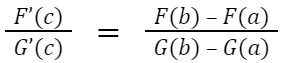## Rolle’s Mean Value Theorem

Rolle’s Mean Value Theorem, also known as RMVT, is a special case of the MVT which can be stated as mentioned below.

Let F(x) be a real-valued function defined on the closed interval [a, b] such that:

• F(x) is continuous on the closed interval [a, b].
• F(x) is differential on the open interval (a, b).
• If F(a) = F(b); Then, there exist a real number c ∈ (a, b) {i.e. a < c < b} such that F’(c) = 0.

## Lagrange’s Mean Value Theorem

Lagrange’s Mean Value Theorem, also known as LMVT, is an extension of the MVT which can be understood as mentioned below.

Let F(x) be a real-valued function defined on the closed interval [a, b] such that:

• F(x) is continuous on the closed interval [a, b].
• F(x) is differential on the open interval (a, b).

Then, there exist a real value c ∈ (a, b) {i.e. a < c < b} such that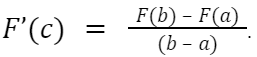## Mean Value Theorem for Integrals

According to the MVT for Integrals, it is stated that if a function F(x) is continuous on the closed interval [a, b], then there exists some real value c ∈ (a, b) {i.e. a < c < b} such that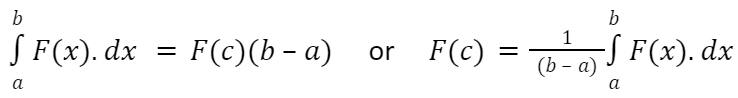The direct consequence of the Calculus’ first fundamental theorem and the MVT is the MVT for Integrals.

## Mean Value Theorem Examples

Let’s discuss about some of the illustrative examples of mean value theorem.

Example-1: Verify the Rolle’s theorem for the function F(x) = x2 – 5x + 6 on the interval [2, 3].

Solution:

Given that, F(x) = x2 – 5x + 6 ———-(1)

? F(2) = (2)2 – 5(2) + 6 = 0

? F(3) = (3)2 – 5(3) + 6 = 0

Now, since a polynomial function is everywhere differentiable and so continuous also.

Therefore,

? F(x) is continuous on [2, 3]. ?F(x) is differentiable on (2, 3).

=> F(2) = F(3) = 0.

Thus, all the conditions of Rolle’s Theorem are satisfied.

Now, we have to show that there exists some c ∈ (2, 3) such that F’(c) = 0.

We have F(x) = x2 – 5x + 6.

? F’(x) = 2x – 5

Since, F’(x) = 0

?2x – 5 = 0 ? x = 5/2 = 2.5

Thus, 2.5 ∈ (2, 3) such that F’(2.5) = 0.

Hence, Rolle’s Theorem is satisfied and verified.

Example-2: Find the value of c, if the function F(x) = x2 – 7x + 12 satisfies the mean value theorem on the interval [3, 4].

Solution:

Since, the function F(x) = x2 – 7x + 12 satisfies the mean value theorem on the interval [3, 4].

Therefore, F(x) is continuous on [3, 4] and F(x) is differentiable on (3, 4).

Given that, F(x) = x2 – 7x + 12 ———-(1)

? F’(x) = 2x – 7

Also,

? F(3) = (3)2 – 7(3) + 12 = 0

? F(4) = (4)2 – 7(4) + 12 = 0

Now, since? F’(c) = [F(4) – F(3)]/(4 – 3)

? 2c – 7 = 0 ? c = 7/2 = 3.5

Therefore, 3.5 ∈ (3, 4) such that F’(3.5) = 0.

Answer: The value of c is equal to 3.5.

## Picked For You

Furthermore, these are some topics which might interest you: